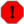# ST_CollectionExtract

## Name

ST_CollectionExtract — Given a (multi)geometry, return a (multi)geometry consisting only of elements of the specified type.

## Synopsis

``` geometry ST_CollectionExtract ( ``` geometry collection , integer type ``` ) ``` ;

## Description

Given a (multi)geometry, returns a (multi)geometry consisting only of elements of the specified type. Sub-geometries that are not the specified type are ignored. If there are no sub-geometries of the right type, an EMPTY geometry will be returned. Only points, lines and polygons are supported. Type numbers are 1 == POINT, 2 == LINESTRING, 3 == POLYGON.

Availability: 1.5.0Prior to 1.5.3 this function returned non-collection inputs untouched, no matter type. In 1.5.3 non-matching single geometries result in a NULL return. In of 2.0.0 every case of missing match results in a typed EMPTY return.When specifying 3 == POLYGON a multipolygon is returned even when the edges are shared. This results in an invalid multipolygon for many cases such as applying this function on an ST_Split result.

## Examples

```-- Constants: 1 == POINT, 2 == LINESTRING, 3 == POLYGON
SELECT ST_AsText(ST_CollectionExtract(ST_GeomFromText('GEOMETRYCOLLECTION(GEOMETRYCOLLECTION(POINT(0 0)))'),1));
st_astext
---------------
MULTIPOINT(0 0)
(1 row)

SELECT ST_AsText(ST_CollectionExtract(ST_GeomFromText('GEOMETRYCOLLECTION(GEOMETRYCOLLECTION(LINESTRING(0 0, 1 1)),LINESTRING(2 2, 3 3))'),2));
st_astext
---------------
MULTILINESTRING((0 0, 1 1), (2 2, 3 3))
(1 row)
```